Next: 6.3 Analytical Comparison of Up: 6.2 Modeling BioFETs Previous: 6.2.5 Debye-Hückel Model

Subsections

## 6.2.6 Buffers and Ionic Strength

Normally, the experiment is carried out in a so called buffer solution. There are several reasons for this. For instance, enzyme reactions are very sensitive to the local temperature, the local substrate concentration, and also to their chemical environment (e.g. pH). In this case the buffer fulfills the function of stabilizing the pH of the solution at a certain point and thus keeping the enzyme activity at its maximum. If DNA is going to be hybridized6.3, the ions in the buffer gather around the single DNA strands and screen partially the DNA charge. The repulsion between the two negatively charged single DNA strands is reduced and they can approach each other close enough to enable the hybridization reaction.

The utilization of buffers is a path way to control the chemical properties of the environment in which the chemical reaction is conducted. Therefore, buffers are also a significant ingredient in the description of BioFETs and a method to calculate the ion concentrations and the ionic strength for an arbitrary buffer will be given. Beynon and Easterby published a book about buffer solutions, giving an exhaustive and easy introduction into this topic .

### 6.2.6.1 Temperature Effects on Buffers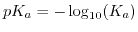is, analogously to the definition of the pH of a solution, for the reaction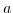. Since the equilibrium constant of a buffer is determined by the laws of thermodynamics, it has to depend on temperature. This is described by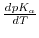, the change ofwith temperature. The logarithmized equilibrium constant depends on the applied buffer and can exhibit positive or negative values, or even being close to zero. Therefore,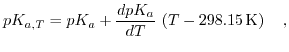(6.9)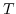denotes the temperature andrepresents the thermodynamicvalue for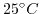.

### 6.2.6.2 Debye-Hückel Relationship

Biological sensors are typically employed at a certain pH. The pH can be adjusted by tritration and monitored by measurement. Hence, pH is an input parameter for the simulation. Starting with a given pH, the buffer ion concentrations can be calculated including temperature effects and the ionic strength of the solution.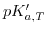determines the buffer ion concentrations but also depends on the ionic strength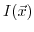and temperature. Since the ionic strength also depends on the buffer ion concentration, this nonlinear equation system has to be solved self-consistently. The effect of the ionic strengthon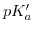can be compressed in one relation (also known as the Debye-Hückel relationship):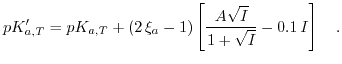(6.10)is termed as modified, working, or practicalvalue,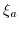is the charge on the conjugate acid species andis a temperature dependent constant.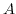is normally around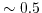(at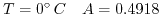and at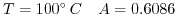). Furthermore,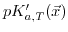is a function of position, because the ionic strength exhibits a position dependence, while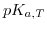is only related to temperature.

### 6.2.6.3 Henderson-Hasselbach Equation

The Henderson-Hasselbach equation connects the relative concentrations of acid and base and theof the conjugate acid/base pair to the pH of the electrolyte,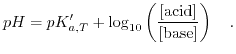(6.11)

On account of the spatial coordinate dependence of all input quantities, the local pH is also a function of position. In the vicinity of the interface the pH value will deviate from the pH value in the bulk electrolyte. Remembering that the ion concentrations are also related to the local potential via the Poisson-Boltzmann equation, further complicates the situation. In order to include all effects one has to employ a numerical approach and solve the equations in a self-consistent manner. A feasible algorithm works as follows:
1. Define the temperatureand the bulk pH value.
2. Define a buffer. Normally there is already one specified from an experiment. If none is available, choose a buffer with anear to the required pH.
3. Correct thevalues to the given temperature.
4. Calculate the concentrations of the conjugate base and acid via the Henderson-Hasselbach equation.
5. Calculate the ionic strength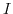of the buffer, including counter ions.
6. Calculatefromwith the ionic strength from the step before.
7. Return to Step 4 and calculate the ionic strengthagain, but this time with the refinedvalues.
8. Calculate thevalues again with the new ionic strength from the step before.
9. Repeat Step 7 and Step 8 until convergence is reached. Typically four cycles are enough to reach a relative error of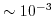.

### 6.2.6.4 PBS as an Example

In order to further clarify the procedure, how to calculate the ionic strength and the different ion concentrations in the buffer, the procedure for a PBS will be presented. PBS contains orthosphoric acid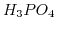and exhibits three dissociation reactions: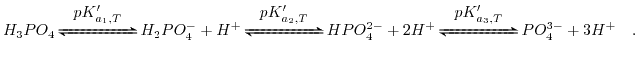(6.12)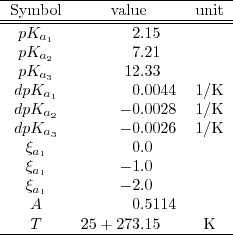Applying the Henderson-Hasselbach equation to (6.13) and utilizing charge conservation to gain the sodium concentration leads to the following set of equations: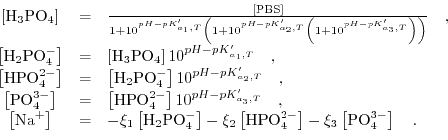(6.13)

Here,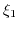,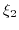, and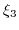are the valencies of the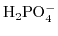,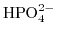, and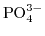, respectively, and the concentration of the PBS and the pH value in the bulk electrolyte is kept fixed. The iteration procedure given before with the values in Table 6.1 yields the ion concentrations depicted in Fig. 6.4, which illustrates the buffer ion concentrations and the ionic strength as a function of pH atand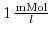. The ionic strength rises strongly for small pH values (high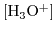concentration) and high pH values (high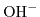concentration). Furthermore, the influence of the valence on the ionic strength is very pronounced due to the quadratic dependence in (6.9).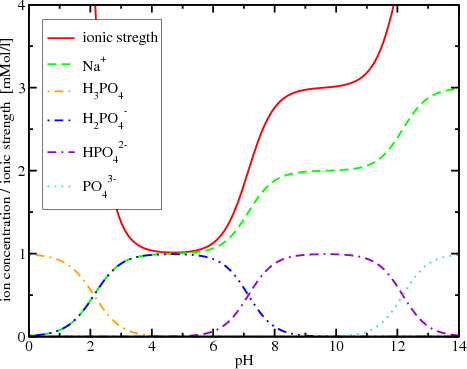#### Footnotes

... hybridized6.3
Nucleic acid hybridization is the process of joining two complementary strands of DNANext: 6.3 Analytical Comparison of Up: 6.2 Modeling BioFETs Previous: 6.2.5 Debye-Hückel Model

T. Windbacher: Engineering Gate Stacks for Field-Effect Transistors